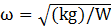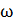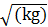### Sample Problem

Fill in the blank with either “directly” or “inversely”.

(1)    Area of an equilateral triangle: A=(√3s2)/4

A is proportional to s.

(2)    Average speed: r=d/t

R is proportional to t.

(3)    Surface area of a sphere: S=4πr2

S is proportional to r.

(4)    Free vibrations:is proportional to W.

#### Solution

(1)    The constant is multiplied to s2 to yield A; √3/4 = k → directly

(2)    The constant divided by t yields average speed; d=k → inversely

(3)    The constant is multiplied to r2 to yield S; 4π = k → directly

(4)    The constant divided by W yields;=k → inversely